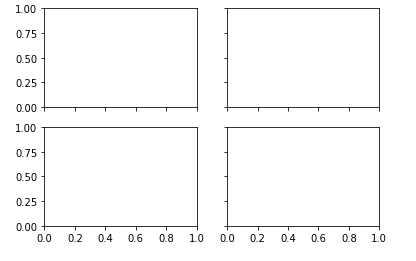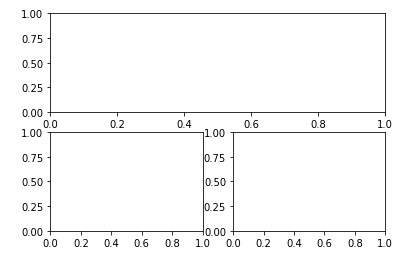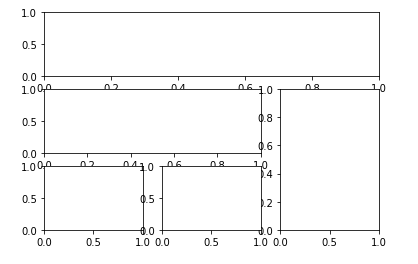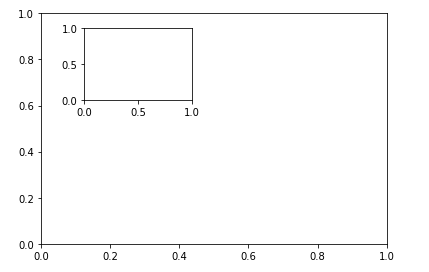## matplotlib绘制图形入门操作：创建画布／调整子图／创建“AXES轴域”/调整子图间的距离/设置XY轴刻度及刻度名称／XY轴标题／图片标题_请在创建一个画布,画布上有1*3个子图,分别是区间[-5,5]上的一次函数图像、二次函_牛奶没法用的博客-程序员宅基地

（1）画布figure可以创建一张或者多张（每张画布是可以“单独”保存）
（2）一张画布上可以创建很多个子图subplot，并根据自己的需求对子图的位置进行部署
（3）每张子图都可以绘制一组数据或者多组数据
（4）对数据的表述，个人选择可以分成：数据描述图形（线图，条形图等），数据分布图形（直方图，饼图，文字云，散点图等）

【疑问】有一个概念Axes“域”，个人认为这个“域”可以理解成一对XY轴，我们可以在一张画布上添加多个“域”（看上去好像多个子图一样），具体有什么实际上区别不明白，之后如果学习到了会更新这个内容

## 1. 创建画布：`plt.figure()`

figure(num=None, figsize=None, dpi=None, facecolor=None, edgecolor=None, frameon=True)

（1）num:图像编号或名称，数字为编号 ，字符串为名称
（2）figsize:指定figure的宽和高，单位为英寸；
（3）dpi参数指定绘图对象的分辨率，即每英寸多少个像素，缺省值为80
（4）facecolor:背景颜色
（5）edgecolor:边框颜色
（6）frameon:是否显示边框

``````# 创建画布
import matplotlib.pyplot as plt

# 创建一个画布，画布序列号为1
fig1=plt.figure()
# 同时创建第二个画布：指定序列号为3；尺寸宽4高3；背景色为蓝（如果不指定序列号则自动增加为2）
fig3=plt.figure(num=3,figsize=(4,3),facecolor='blue')
``````

## 2. 创建子图：

（1）固定行列分布的子图组布局（是否可以修改子图大小形状不确定）：plt.subplots()
（3）创建单个子图在一个固定行列分布的网格组中，子图形状任意布局：plt.subplot2grid()

• `plt.subplots()`的方法

plt.subplots(nrows=1, ncols=1, sharex=False, sharey=False, squeeze=True, subplot_kw=None, gridspec_kw=None, **fig_kw) ：

（1）nrows=1, ncols=1：制作几行列的子图组
（2）sharex=False, sharey=False：子图组中XY轴的刻度是否一致，默认不一致

（1）fig : Figure
（2）ax : axes.Axes object or array of Axes objects.

``````# 创建一个figure和一个2行2列的子图组
fig,axes=plt.subplots(2,2)
# 划分子图
ax1=axes[0,0]
ax2=axes[0,1]
ax3=axes[1,0]
ax4=axes[1,1]

# 另一种书写方式
f, ((ax11, ax12), (ax13, ax14)) = plt.subplots(2, 2)
``````• `plt.figure().add_subplot()`的方法与`plt.subplot()`的方法

.add_subplot(nrows, ncols, index, **kwargs) 和.subplot(nrows, ncols, index, **kwargs) 函数功能是一样的，区别在于：

``````import matplotlib.pyplot as plt

fig = plt.figure()

# 使用plt.subplot()创建一个：上面一个图，下面两个图的布局
plt.subplot(2,1,1)				# 位置上，相当于占了一整行，“两列”

plt.subplot(2,2,3)				# 第二行，第一列
plt.subplot(2,2,4)				# 第二行，第二列

plt.show()
``````• `plt.subplot2grid()`方法

plt.subplot2grid（shape，loc，rowspan = 1，colspan = 1，fig = None，** kwargs ）的主要参数解释如下：
a. shape : 制作几行几列的子图布局
b. loc : 当前子图的位置在哪行哪列
c. rowspan : 跨几行
d. colspan : 跨几列

``````import matplotlib.pyplot as plt

# 创建一个3行3列的子图格，该子图位于0行0列的位置，跨3列
ax1 = plt.subplot2grid((3, 3), (0, 0), colspan=3)  # stands for axes
# 创建一个3行3列的子图格，该子图位于1行0列的位置，跨2列
ax2 = plt.subplot2grid((3, 3), (1, 0), colspan=2)
# 创建一个3行3列的子图格，该子图位于1行2列的位置，跨2行（1行中的0和1索引的列被ax2填充）
ax3 = plt.subplot2grid((3, 3), (1, 2), rowspan=2)
ax4 = plt.subplot2grid((3, 3), (2, 0))
ax5 = plt.subplot2grid((3, 3), (2, 1))
``````• `plt.figure().add_axes()`方法

left, bottom表示左边以及下边的起始位置，width, heigh表示宽高延伸原fig的比例。

``````import matplotlib.pyplot as plt

# 首先需要创建一个画布的对象
fig = plt.figure()

# 子区域从figure 10%的位置开始绘制, 宽高延伸figure的80%
left, bottom, width, height = 0.1, 0.1, 0.8, 0.8
ax1 = fig.add_axes([left, bottom, width, height])  # main axes

# 子区域从figure 20%+60% 的位置开始绘制, 宽高增长figure的25%
ax2 = fig.add_axes([0.2, 0.6, 0.25, 0.25])  # inside axes
``````## 3. 调整子图间的距离：`plt.subplots_adjust()`

subplots_adjust（left = None，bottom = None，right = None，top = None，wspace = None，hspace = None ）

（1）left = 0.125 ：the left side of the subplots of the figure
（2）right = 0.9：the right side of the subplots of the figure
（3）bottom = 0.1：the bottom of the subplots of the figure
（4）top = 0.9：the top of the subplots of the figure
（5）wspace = 0.2 ： the amount of width reserved for space between subplots, expressed as a fraction of the average axis width
（6）hspace = 0.2：the amount of height reserved for space between subplots, expressed as a fraction of the average axis height

## 4. 设置XY轴刻度及刻度名称／XY轴标题／图片标题

• XY轴刻度及刻度名称

``````months = range(12）
month_names = ["Jan", "Feb", "Mar", "Apr", "May", "Jun", "Jul", "Aug", "Sep","Oct", "Nov", "Dec"]

# 只有画布
plt.xticks(months,month_names)
plt.yticks([0.10, 0.25, 0.5, 0.75],['10%', '25%', '50%', '75%'])

# ax=plt.subplot()
ax.set_xticks(months)		# 设置X轴刻度值
ax.set_xticklabels(month_names)		# 匹配X轴刻度名字

ax.set_yticks([0.10, 0.25, 0.5, 0.75])		# 设置Y轴刻度值
ax.set_yticklabels(['10%', '25%', '50%', '75%'])		# 匹配Y轴刻度名
``````
• 设置XY轴名称

``````# 只有画布
plt.xlabel("Months")
plt.ylabel('Conversion')

# 创建了子图对象或者“域”对象
ax.set_xlabel("Months")
ax.set_ylabel('Conversion')
``````
• 设置标题

``````# 只有画布时
plt.title('My Last Twelve Years of Coffee Drinking')

# 创建了子图对象或者“域”对象
ax.set_titel("My Last Twelve Years of Coffee Drinking")
``````

### 从Delphi 7升级到Delphi XE_delphixe和delphi7_ppayun的博客-程序员宅基地

Delphi 7和XE中间有好几个版本，可都不太流行，一是因为Delphi有些边缘化，二是7在XP环境下是近乎完美的版本。市场上关于delphi的书越来越少，提供从7升级到XE介绍的资料几乎没用。这里我试着列举一下7和XE的差异和注意事项，给需要升级的朋友一些线索。1. 7和XE是可以共存的，7的工程文件是.dpr，XE的是.dproj，用XE打开dpr文件会提示升级，自动创建新的项目文件。X_delphixe和delphi7

### 炒鸡简单的bfs_分享vn1.9.13(一款炒鸡强大简单的剪辑软件)_soul,,,明灵的博客-程序员宅基地

Dungeon MasterYou are trapped in a 3D dungeon and need to find the quickest way out! The dungeon is composed of unit cubes which may or may not be filled with rock. It takes one minute to move one un..._分享vn1.9.13(一款炒鸡强大简单的剪辑软件)

### RNN模型_Houser_qihao的博客-程序员宅基地

RNN模型我们先来看一个RNN经典结构，图1展示了一个典型按时间展开后的RNN结构。从图1可以看出，RNN在每一个时刻都有一个输入Xt，然后根据当前节点的状态At计算输出值ht，而At是根据上一时刻的状态At-1和当前的输入Xt共同决定的。和卷积神经网络卷积核或池化核的参数共享类似，这里RNN结构中的参数在不同时刻中也是共享的。1.2.1 RNN前向传播过程图2 RNN的前..._rnn模型

### BindingSource的使用范例_QQ1422078449的博客-程序员宅基地

public partial class Form1 : Form { private DataSet gDataSet = GetDS(); public Form1() { InitializeComponent(); // Set the data sourc

### Element-ui input框效验_XLLcsdn的博客-程序员宅基地

<template> <div class="info" <el-form :model="batch" :rules="rules" ref="batch"> <el-form-item label="审核意见：" prop="auditOpinion" class="auditOpinion" ref="auditOpinion"> ...

### 十进制转二进制原理_假装会飞的博客-程序员宅基地

1. 十进制整数转二进制假设十进制正整数值为A，对应的二进制数为abcde。众所周知，二进制数换算为十进制的公式如下：A = a(2^4) + b(2^3) + c(2^2) + d(2^1) + e(2^0)所以咱们只要把a,b,c,d,e都取出来就能得到二进制的值。又 A = 2 * (a(2^3) + b(2^2) + c(2^1) + d(2^0)) + e所以A除以2即能得到整数位a(2^3) + b(2^2) + c(2^1) + d(2^0) 和余数e上边得到的整数a_十进制转二进制原理

### 7 客户端认证方式 之 client_secret_jwt_python jwt client_secret client_id_markix的博客-程序员宅基地

`client_secret_jwt`方式就是利用 `JWT` 进行认证。请求方和授权服务器，两者都知道客户端的 `client_secret`，通过相同的 `HMAC` 算法（对称签名算法）去加签和验签 `JWT` ，可以达到客户端认证的目的。_python jwt client_secret client_id

### python注册表_Python模块——_winreg操作注册表 | 学步园_土盐的博客-程序员宅基地

From:http://blog.sina.com.cn/s/blog_4b5039210100gmsb.html用python操作修改windows注册表，显然要比用C或者C++简单。通过python操作注册表主要有两种方式，一种是通过python的内置模块_winreg，另一种方式就是Win32Extension For Python的win32api模块。这里主要简单看看用内置模块_winr..._dir, type = _winreg.queryvalueex(key, shell_folder_name)dir, type = _winreg.